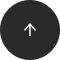MySQL

# How to Calculate a Square Root in SQL

SQRT

#### Problem:

You want to find the square root of a number.

#### Example:

You want to compute the square root of all numbers in the column `number` from the table `data`.

number
9
2
1
0.25
0
-4

#### Solution 1:

```SELECT
number,
SQRT(number) AS square_root
FROM data;
```

The result is:

numbersquare_root
93
21.4142135623731
11
0.250.5
00
-4error

#### Discussion:

To compute the square root of a number, use the `SQRT()` function. This function takes a `number` as its argument and returns the square root.

Note that there is no real square root from a negative number (imaginary numbers aren't supported) – hence the error.

Also, for most numbers (e.g., 2, 2.5, 3, 3.2 etc.) the square root is an irrational number – in the square_root column you won't see the exact results, only the first several digits of their decimal expansion.

Also, for most numbers (e.g., 2, 2.5, 3, 3.2 etc.) the square root is an irrational number – in the `square_root` column you won't see the exact results, only the first several digits of their decimal expansion.

#### Recommended articles: